# How to add numbers (part 2)

14 Nov 2012

Last time, I explained how adders work in CPUs, and one nice trick for speeding them up. Be sure to read part 1 before diving into this!

## Generation and propagation

In 1958, some sharp fellows named Weinberger & Smith hit the carry ripple problem from a different angle. Even if you don’t know what a column’s carry-in will be yet, you can make some assumptions about what will happen:

A B Cout
000
01Cin
10Cin
111

If both inputs are 0, the carry will definitely be 0, so the carry is “killed”. If both are 1, the carry will definitely be 1, so a carry is “generated”. Both of these cases are the same whether the carry-in is 0 on 1. But if only one of the inputs is 1, then we’ll only have a carry-out if we had a carry-in, so a carry is “propagated”.

We can use “G” to mean a 1-bit adder would generate a carry by itself, and “P” to mean it will propagate its incoming carry.

G = A⋅B
P = A⊕B

So, for any column, the carry-out will be 1 if either “G” is 1 (it generates a carry), or “P” is 1 (it propagates a carry) and the carry-in is 1.

Cout = G + P⋅Cin

For the lowest bit, if we substitute G and P into the above equation, we get:

Cout = A⋅B + (A⊕B)⋅Cin

which is equivalent to our original carry-out equation:

Cout = A⋅B + A⋅Cin + B⋅Cin

The fun comes when you consider the second bit. It will have a carry-out if it generates one, or it propagates one and the lowest bit generated one, or it propagates one and the lowest bit propagates one and the carry-in was 1.

C0 = G0 + P0⋅Cin
C1 = G1 + P1⋅G0 + P1⋅P0⋅Cin
C2 = G2 + P2⋅G1 + P2⋅P1⋅G0 + P2⋅P1⋅P0⋅Cin
...

## Parallel (in small doses)

This series can go on indefinitely. If we compute a G and P for each column, then we can compute the carry bit for a column N by making an `OR` gate with N + 2 inputs, each of which is a G and a string of Ps, with the last `AND` gate having N + 1 inputs. We could compute each carry bit in 3 gate delays, but to add 64 bits, it would require a pile of mythical 65-input `AND` and `OR` gates, and a lot of silicon.

It’s more feasible for small adders, like 4 or 8 bits at a time. Here’s a sample two-bit adder that computes the two carry-out bits in parallel, by computing P and G first: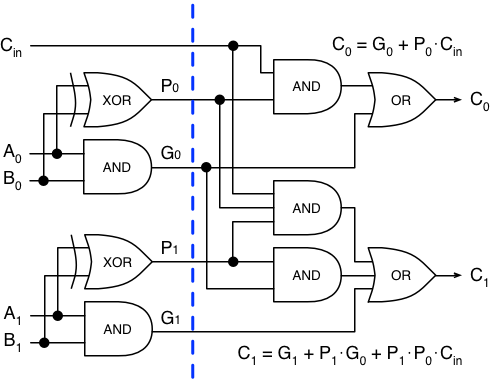That circuit is already a bit intimidating to look at, so I didn’t show the sum bits, but remember that the sum bit is

S = A⊕B⊕Cin

or, using P:

S = P⊕Cin

So the sum for any column is just an `XOR` of the carry-in bit and the P bit that we already computed for our carry-out. That adds one more gate, for a total of 4 gate delays to compute the whole 2-bit sum.

If we built a set of 4-bit adders this way – assuming a 6-way `OR` gate is fine – our carry-select adder could add two 64-bit numbers in 19 gate delays: 3 for all of the carries to be generated, and 16 for the muxes to ripple down. These ripples now account for almost all of the delay.

## Kogge-Stone

In 1973, probably while listening to a Yes or King Crimson album, Kogge and Stone came up with the idea of parallel-prefix computation. Their paper was a description of how to generalize recursive linear functions into forms that can be quickly combined in an arbitrary order, but um, they were being coy in a way that math people do. What they were really getting at is that these G and P values can be combined before being used.

If you combine two columns together, you can say that as a whole, they may generate or propagate a carry. If the left one generates, or the left one propagates and the right one generates, then the combined two-column unit will generate a carry. The unit will only propagate a carry bit across if both columns are propagating. It looks like this:

Gunit = G1 + P1⋅G0
Punit = P1⋅P0

In a circuit, it adds 2 gate delays, but can be used to combine any set of P and G signals that are next to each other, and even to combine some P and G signals that are already combined. On the right, below, is the symbol we’ll use to represent this combining operation from now on: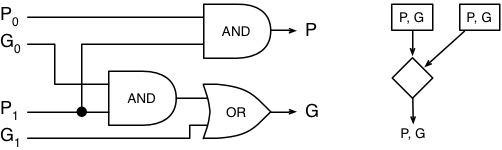Any time we can do a recursive combination like this, we’re in log-scale country. This is the country where cowboys ride horses that go twice as far with each hoofstep. But seriously, it means we can compute the final carry in an 8-bit adder in 3 steps.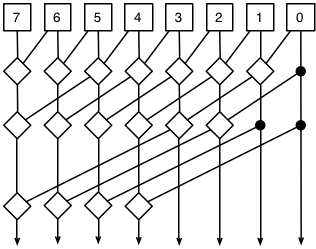Wait, what? Well, the numbers at the top represent the computed P and G bit for each of the 8 columns of our 8-bit adder. The diamonds combine two adjacent sets of columns and produce a new combined P and G for the set. If this works, at the bottom, each arrow should represent the combined P and G for that column and every column to its right.

Look at the line on the far left, and trace it back up. It combines the lines from 7 and 3, and as we trace that up again, each of those combines two more units, and then again to cover all 8 columns. The same path up should work for each column.

There are lots of wires/connections because we need to compute the combined P and G for each column, not just the final one. These combined P and G values represent the combined value for each set of columns all the way to the right edge, so they can be used to compute the carry-out for each column from the original carry-in bit, instead of rippling:

Cn = Gn-combined + Pn-combined⋅Cin

The sum bit can still be computed with a final `XOR`, using the original (not combined) P and the carry bit to its immediate right:

Sn = Pn⊕Cn-1

This final step adds three gates to the end of each column. As we saw above, each combining operation is two gates, and computing the original P and G is one more. For this 8-bit adder, which uses three combining steps, we wait 1 + 3⋅2 + 3 = 10 gate delays for the result. For a 64-bit adder, we need 6 combining steps, and get our result in 16 gate delays!

The Kogge-Stone adder is the fastest possible layout, because it scales logarithmically. Every time we add a combining step, it doubles the number of bits that can be added. It’s so efficient that 25% of the delay in our 64-bit adder will be the setup and final computation before and after the combining phase. The only real flaw is that the number of wires gets a little crazy – the 8-bit adder is already filled with cross-connections, and that gets so much worse in the 64-bit version that I’m not going to try to draw it. It might even monopolize a lot of the chip space if we tried to build it.

Luckly, there’s a compromise that adds a few steps but removes a lot of the wires.

## Brent-Kung

In 1982, Brent & Kung described this clever modification, which just computes the left-most column in a binary tree, and then fills in the intermediate columns in a reverse tree: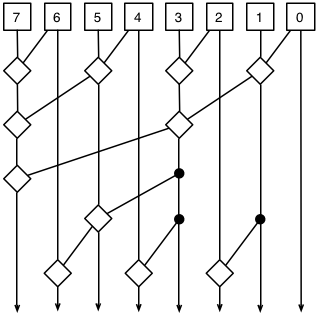If you walk up the tree from bottom to top on any column, it should still end up combining every other column to its right, but this time it uses far fewer connections to do so. A nice paper from 2007 compares several adder strategies and decides that this one is the most energy-efficient because of the trade-off of speed for simplicity. That is, it can be built easier than the Kogge-Stone adder, even though it has nearly twice as many combination steps in it. For our 64-bit adder, we’d have 11 steps, for 1 + 11 ⋅ 2 + 3 = 26 gate delays. (This is more than our best-case of 16 for the Kogge-Stone adder, and a bit more than our naive-case of 24 with the carry-select adder.)

One potential problem is “fan-out”, which means one outgoing signal is being sent to several other gates as inputs. Electronics people would say one gate is “driving” a bunch of other gates, and this is bad, because the current gets split several different ways and diluted and weakened, just like water through a fork in a pipe. You can see this especially in column 3. A Brent-Kung adder will actually turn the joints (that I’ve marked with black circles) into buffers, or gates that don’t do anything. That reduces the fan-out back to 2 without slowing anything down.

## Hybrid

One thing you might have spotted with your eagle eye is that the Brent-Kung adder doesn’t slow down the left-most column, which generates the final carry- out bit. So if we were to combine this strategy with the carry-select strategy from last time, our carry bits could start rippling across the adder units before each unit finishes computing the intermediate bits. Hmm.

An `n`-bit Brent-Kung adder will be able to generate the carry-out bit in log2(n) steps, using 2 gates per step, with an additional gate delay for computing P and G for each bit, and two extra gate delays to compute the carry-out from the combined P/G.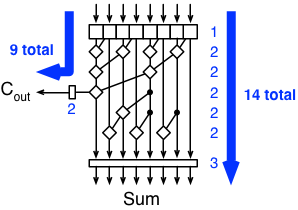The full sum will take an extra log2(n) - 1 steps, and an extra gate to do the P⊕Cin operation.

When a carry-select adder is used with `k` units, the ripple delay is `k` plus the time it takes to get a carry-out from the first unit.

So if we split our 64-bit adder into 8 8-bit Brent-Kung adders, and combine those into a carry-select adder, the 8-bit adders will compute their carry-out bits in 9 gate delays, after which the carry bits ripple through the muxes for 7 gate delays, for a total of 16. The sum bits are available after 14 gate delays, in plenty of time. So we got it down to 16 total, and this time in a pretty efficient way!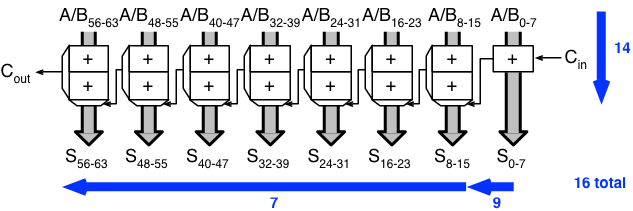Adding numbers: Proof that humans can make anything complicated, if they try hard enough.

There are a bunch of other historical strategies, but I thought these were the most interesting and effective. If you stuck it out through both articles, I’d love to hear your thoughts, ideas, and/or corrections.